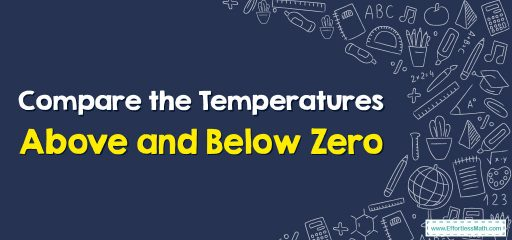# Compare the Temperatures Above and Below Zero

When learning how to compare temperatures above and below zero, it's important to have a solid understanding of the temperature scale being used.The two most commonly used temperature scales are Celsius and Fahrenheit.

In both of these scales, zero represents a key point: for Celsius, zero degrees represents the freezing point of water, while for Fahrenheit, zero degrees represents the temperature achieved by mixing equal parts of ice, water, and salt.

## A step-by-step guide to compare the temperatures above and below zero

It is possible to represent the temperature changes on a number line.

Negative numbers are on the left-hand side of 0.

Positive numbers are on the right-hand side of 0.

The numbers beyond the right-hand side (positive) are always larger than the ones on the left-hand side (negative).

To compare temperature:
The coldest temperatures (the lower number) are on the left-hand side of 0.
The warmest temperatures (the higher numbers) are on the right-hand side of 0.

Comparing temperatures above and below zero can be done in a few different ways, depending on the context and the scale being used.

Here are some general tips for comparing temperatures in each scale:

In Celsius:

• If you’re comparing temperatures above zero, simply compare the numbers as you normally would. For example, 20 degrees Celsius is warmer than 10 degrees Celsius.
• If you’re comparing temperatures below zero, remember that the further below zero a temperature is, the colder it is. For example, -10 degrees Celsius is colder than -5 degrees Celsius.

In Fahrenheit:

• If you’re comparing temperatures above 0°F, again, simply compare the numbers as you normally would. For example, 70°F is warmer than 60°F.
• If you’re comparing temperatures below 0°F, remember that the negative numbers represent temperatures that are progressively colder. So, -10°F is colder than -5°F.

Overall, understanding how to compare temperatures above and below zero is an important skill for a variety of applications, including weather forecasting, scientific research, and cooking.

By understanding the temperature scale being used and keeping in mind the basic principles outlined above, anyone can learn to make accurate temperature comparisons.

### Compare the Temperatures Above and Below Zero – Example 1

Yesterday, at 2:00 AM the temperature was 2 degrees Celsius. And, at 2:00 PM the temperature was 10 degrees Celsius. Did the temperature rise or fall yesterday?
Solution:
10 degrees Celsius is warmer than 2 degrees Celsius. So, the temperature rose.

### Compare the Temperatures Above and Below Zero – Example 2

Noah recorded a temperature of 17 degrees Celsius, and Carol recorded a temperature of 21 degrees Celsius. Did Carol record a temperature that was warmer or cooler?
Solution:
21 degrees Celsius is larger than 17 degrees Celsius. So, the temperature was warmer.

### What people say about "Compare the Temperatures Above and Below Zero - Effortless Math: We Help Students Learn to LOVE Mathematics"?

No one replied yet.

X
30% OFF

Limited time only!

Save Over 30%

SAVE $5 It was$16.99 now it is \$11.99